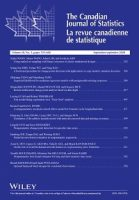# Layman’s abstract for Canadian Journal of Statistics article on Penalized high-dimensional M-quantile regression: From L1 to Lp optimization

Each week, we publish layman’s abstracts of new articles from our prestigious portfolio of journals in statistics. The aim is to highlight the latest research to a broader audience in an accessible format.The article featured today is from the Canadian Journal of Statistics, with the full article now available to read here.

Hu, J., Chen, Y., Zhang, W. and Guo, X. (2021), Penalized high-dimensional M-quantile regression: From L1 to Lp optimization. Can J Statistics. https://doi.org/10.1002/cjs.11597

Studying the whole conditional distribution of a response variable given predictors is of great interest in a heterogeneous regression setting, especially when the dimension of covariates is ultra-high in many research fields. How to detect heterogeneity properly? How do we select the truly significant covariates in ultra-high dimensional data?

The common approach to address heterogeneous data is quantile regression since it allows one to study the quantile structure of the conditional distribution. While quantile regression has a strong intuitive appeal and is robust to outliers, it has three drawbacks: non-differentiability, inefficiency for Gaussian-like error distributions, and difficulty in calculation of a covariance matrix. As an alternative, expectile regression based on an asymmetric L2 norm is developed, which is more sensitive to extreme values than quantile regression. However, when data are homogeneous with some outliers rather than heterogeneous, expectile regression is more affected by those outliers.

In this paper, the authors consider Lp-quantile regression to study the Lp-quantile structure of the conditional distribution, which combines the robustness properties of quantile regression and the sensitivity properties of expectile regression. To deal with high dimensionality, this paper investigates Lp-quantile regression with SCAD and adaptive-LASSO penalties.

The oracle properties, i.e., the sparsity of estimator and asymptotic normality of nonzero coefficients are obtained under these two types of penalties. This work confirms that the proposed penalized high-dimensional Lp-quantile regression steers an advantageous middle course between ordinary penalized quantile and expectile regressions without sacrificing their virtues too much when 1 < p < 2. In real applications, it also suggests from this paper that the choice of p ≥ 1.2 is more reasonable in penalized high-dimensional Lp-quantile regression to reduce variance.